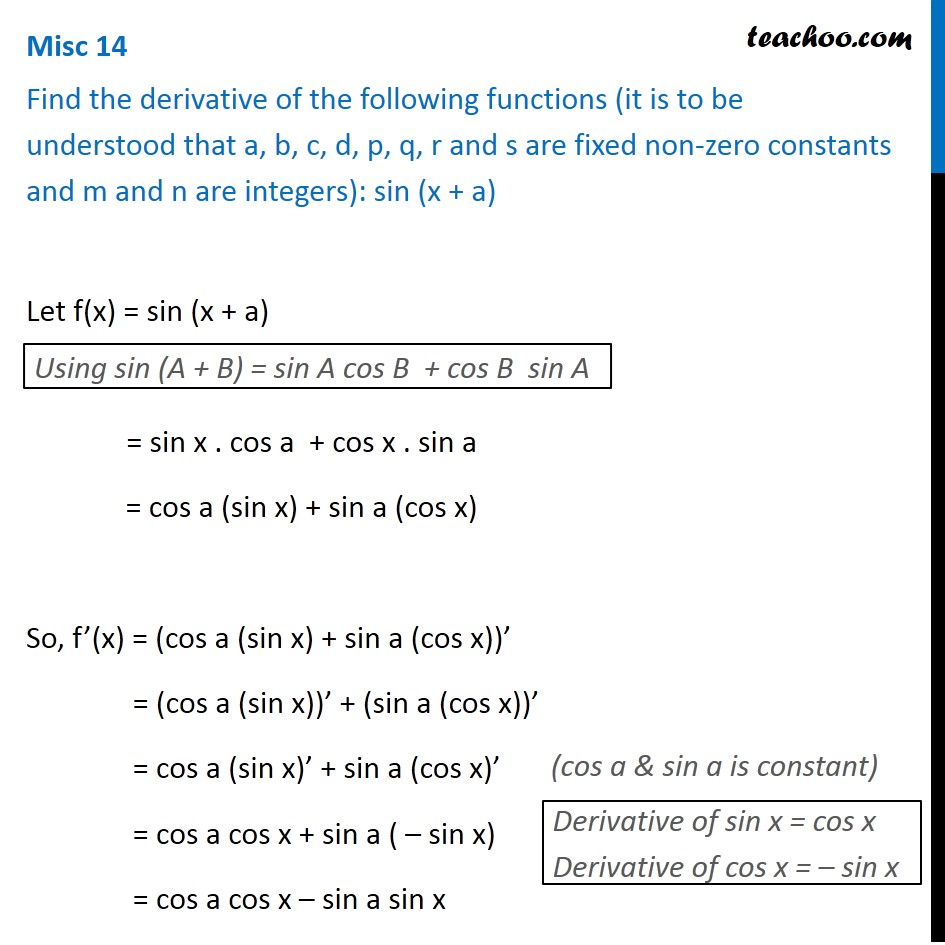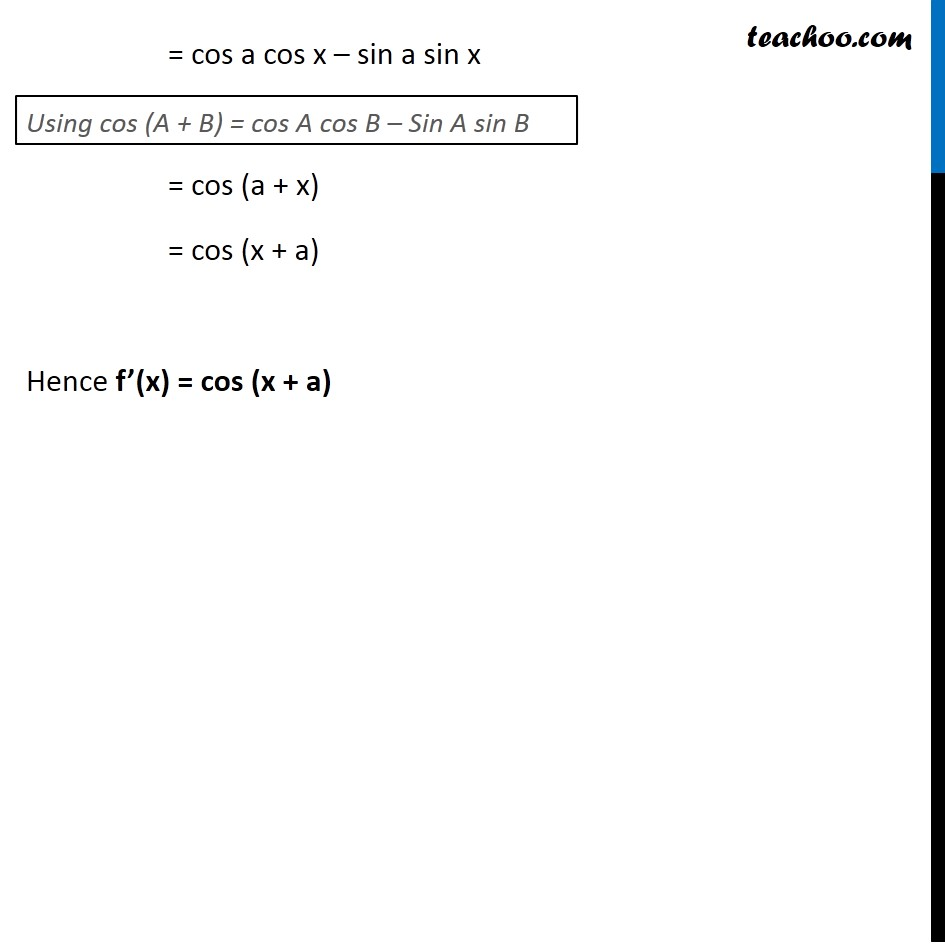Derivatives by formula - sin & cos

Chapter 13 Class 11 Limits and Derivatives
Concept wiseIntroducing your new favourite teacher - Teachoo Black, at only ₹83 per month

### Transcript

Misc 14 Find the derivative of the following functions (it is to be understood that a, b, c, d, p, q, r and s are fixed non-zero constants and m and n are integers): sin (x + a) Let f(x) = sin (x + a) Using sin (A + B) = sin A cos B + cos B sin A = sin x . cos a + cos x . sin a = cos a (sin x) + sin a (cos x) So, f’(x) = (cos a (sin x) + sin a (cos x))’ = (cos a (sin x))’ + (sin a (cos x))’ = cos a (sin x)’ + sin a (cos x)’ = cos a cos x + sin a ( – sin x) = cos a cos x – sin a sin x Derivative of sin x = cos x Derivative of cos x = – sin x = cos a cos x – sin a sin x = cos (a + x) = cos (x + a) Hence f’(x) = cos (x + a) Using cos (A + B) = cos A cos B – Sin A sin B